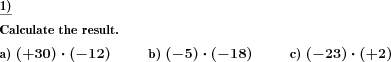Custom math worksheets at your fingertips# Details for problem "Multiplication of negative and positive numbers"

Quickname: 9229

Elementary School, Primary School, Junior High School, Middle School, High School.

## Summary

Multiplication of two negative numbers, training the rules for the product's sign.

## Example## Description

This exercise trains the handling of negative numbers during multiplication. On the one hand, the absolute result must be calculated correctly, and on the other hand, the sign must be determined correctly. The corresponding rules can also be practiced with this activity.

The two factors are determined randomly from a number range that can be specified. At least one factor has a negative sign. It is also possible that both factors are negative. The correct result must be given.

No particular approach to the solution is given or expected. Thus this task is suitable for different calculation methods, also for the solution with the pocket calculator.

The number of problems can be configured.

Download free printable worksheets for this math problem here. The worksheet contains the problems only, the solution sheet includes the answers. Just click on the respective link.

•Worksheet 1Solution sheet with answers
•Worksheet 2Solution sheet with answers
•Worksheet 3Solution sheet with answers

If you can not see the solution sheets for download, they may be filtered out by an ad blocker that you may have installed. If this is the case, please allow ads for this page and reload the page. The solution sheets will then reappear.

• Do these sample worksheets do not really fit?
• Do you need more math worksheets, with a different level of difficulty?
• Would you like to combine different problems on a worksheet and adjust them to your needs?
• As a teacher, you can put together your own worksheets using the automatically generated math problems provided.
With a free initial credit, you can start creating your own math worksheets in a few minutes.

You can try it for free! Register here, to create custom worksheets now!

## Customization options for this problem

Parameter
Possible values
Number of problems
1, 2, 3, 4, 5, 6, 7, 8, 9, 10
Number range
10, 20, 30, 40, 50, 100, 200, 500, 1000, 10000, 100000

## Similar problems

Remark
Description
A fill-in problem: Frames and arrows
In a frames and arrows calculation chain task, the results are to be filled in the blank spaces.
General multiplication of natural numbers
Multiplication problems with defined number rangeDeutsche Version dieser Aufgabe
These informational pages with samples describe math problems that can be combined on custom math worksheets with solutions for home and K-12 school use.
Deutsche Seiten
×